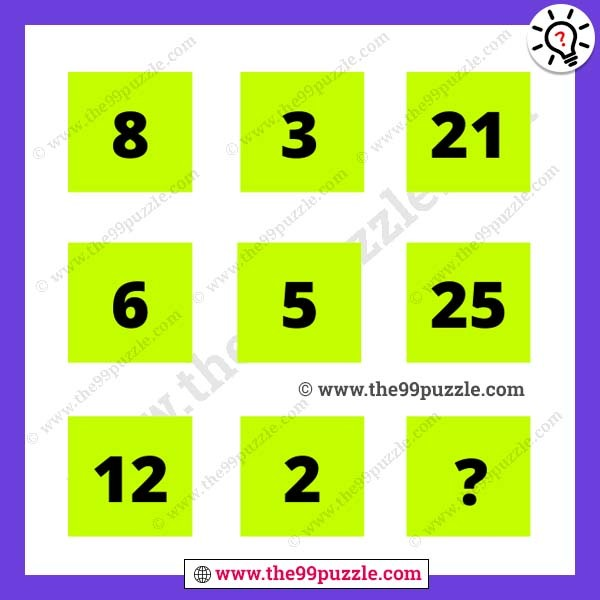# Logical reasoning mathematical puzzle with answer – Puzz214

Can you solve the logical reasoning mathematical puzzle? We are sharing many brain teaser math puzzles for all genius people. Learn more math tricks and shortcut math to solve these math puzzles. Sometimes most genius people fail to answer this type of math reasoning. If you are a competitive aspirant you have to solve all these missing number puzzles. In this puzzle, you see some numbers in the box. So, now find out the math logic and replace the question mark?###### Explanation:

1st row = (8 − 1) × 3 = 7 × 3 = 21

2nd row = (6 − 1) × 5 = 5 × 5 = 25

3rd row = (12 − 1) × 2 = 11 × 2 = 22